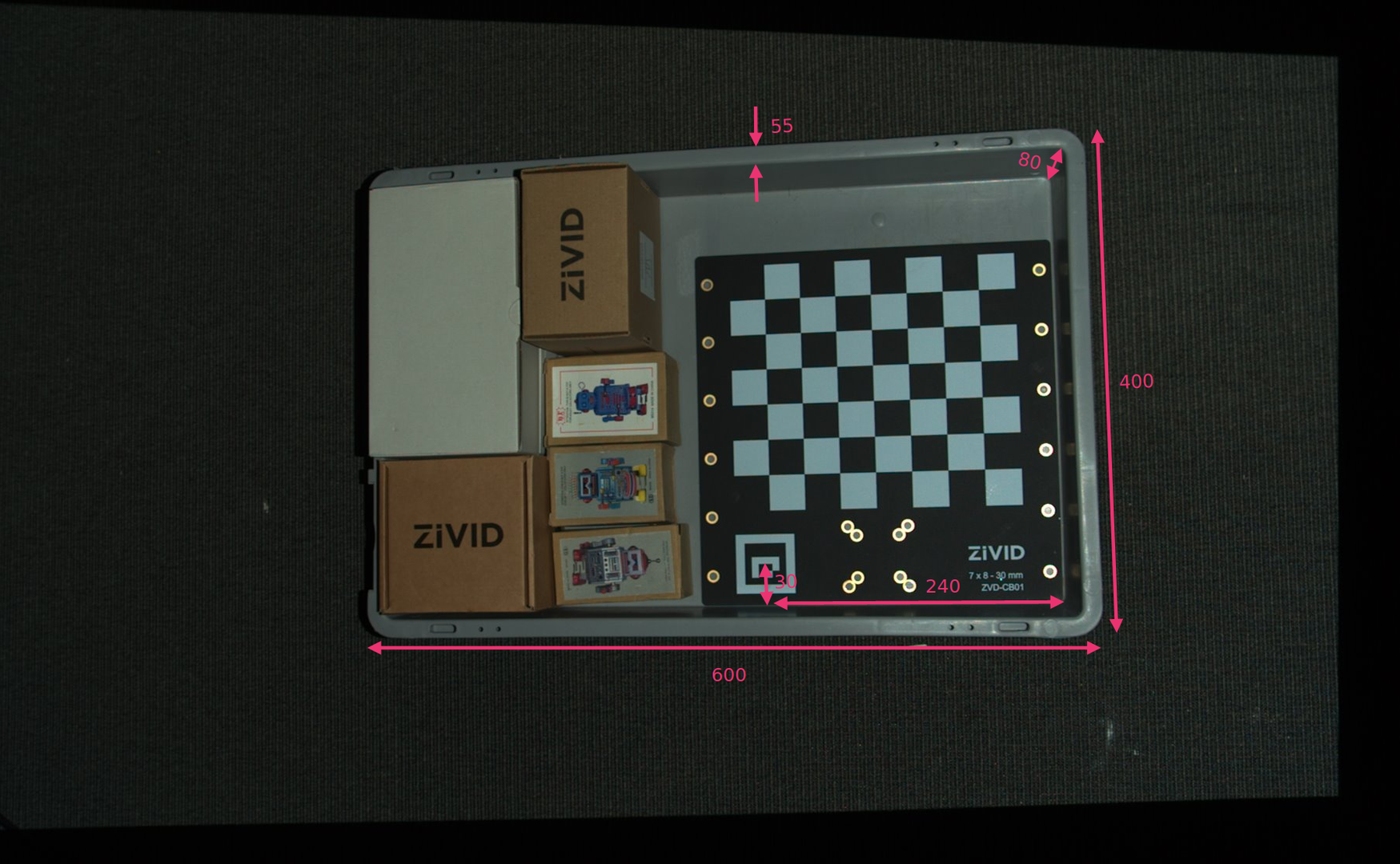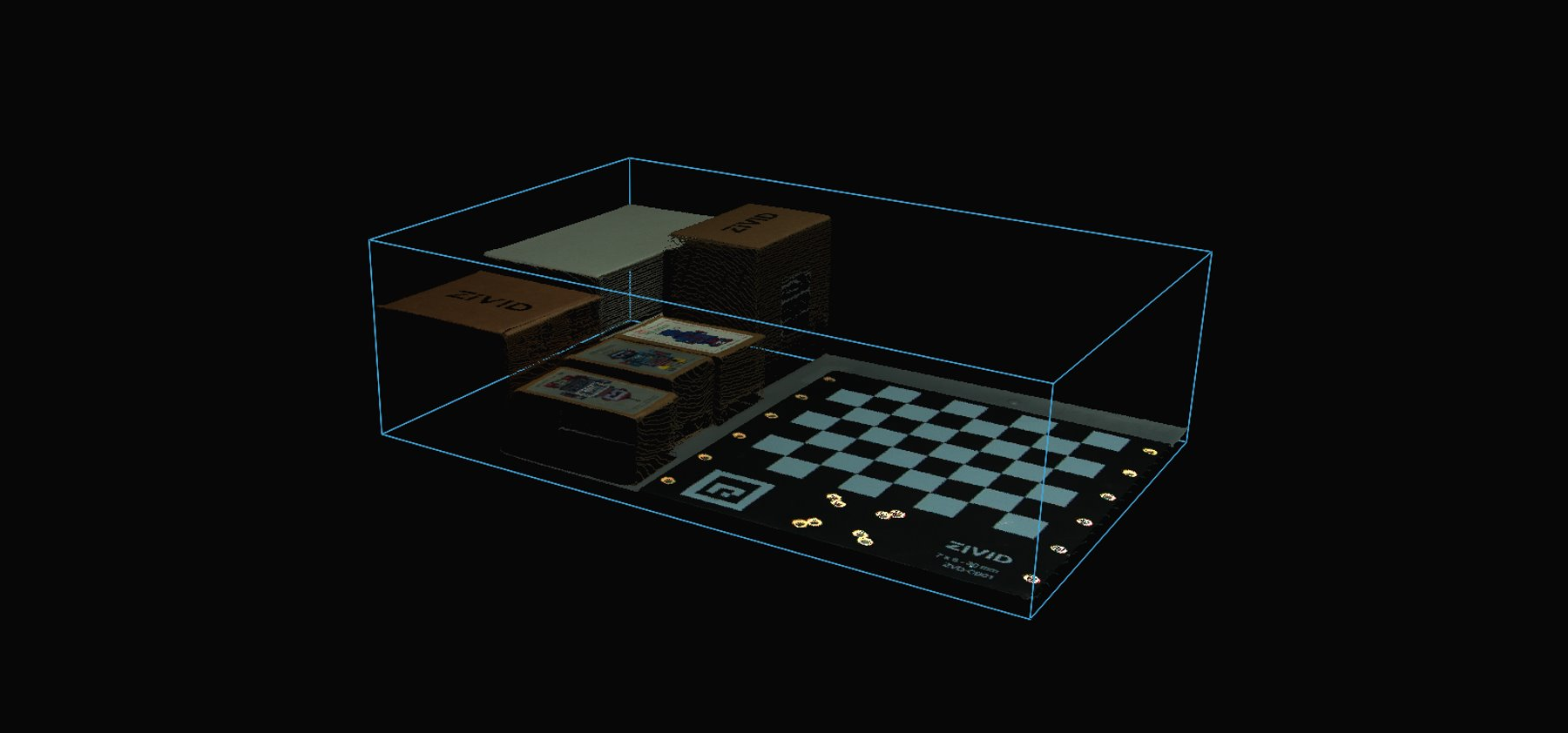# ROI box filter via ArUco marker

This tutorial demonstrates how to filter the point cloud transformed to the ArUco marker coordinate system based on a ROI box given relative to the marker. This results in filtering out all the points that are outside of the bin.

Tip

Zivid calibration board contains an ArUco marker.

This tutorial uses the point cloud of a ArUco marker displayed in the image below.We can open the `original point cloud` in Zivid Studio and inspect it.

Note

The original point cloud is also in Sample Data.

To skip the part about transforming the point cloud, which is also covered in Transform via ArUco marker, go to Filtering based on ROI box.

Transforming the point cloud

First, we load a point cloud of an ArUco marker.

```const auto arucoMarkerFile = std::string(ZIVID_SAMPLE_DATA_DIR) + "/BinWithCalibrationBoard.zdf";
std::cout << "Reading ZDF frame from file: " << arucoMarkerFile << std::endl;
const auto frame = Zivid::Frame(arucoMarkerFile);
auto pointCloud = frame.pointCloud();
```

Since we are using OpenCV, we need to convert the 2D image from the point cloud to OpenCV image format.

```std::cout << "Converting to OpenCV image format" << std::endl;
const auto grayImage = pointCloudToGray(pointCloud);
```

Then we configure the ArUco marker.

```std::cout << "Configuring ArUco marker" << std::endl;
const auto markerDictionary = cv::aruco::getPredefinedDictionary(cv::aruco::DICT_4X4_100);
std::vector<int> markerIds;
std::vector<std::vector<cv::Point2f>> markerCorners;
cv::Ptr<cv::aruco::DetectorParameters> detectorParameters = cv::aruco::DetectorParameters::create();
detectorParameters->cornerRefinementMethod = cv::aruco::CORNER_REFINE_SUBPIX;
```

We then detect the ArUco marker in the 2D image.

```std::cout << "Detecting ArUco Marker" << std::endl;
cv::aruco::detectMarkers(grayImage, markerDictionary, markerCorners, markerIds, detectorParameters);
```

Then we estimate the pose of the ArUco marker.

```std::cout << "Estimating pose of detected ArUco marker" << std::endl;
const auto transformMarkerToCamera = estimateArUcoMarkerPose(pointCloud, markerCorners);
const auto transformCameraToMarker = transformMarkerToCamera.inverse();
```

We then transform the point cloud to the ArUco marker coordinate system.

```std::cout << "Transforming point cloud from camera frame to ArUco marker frame" << std::endl;
pointCloud.transform(transformCameraToMarker);
```

Filtering based on ROI box

Now we define the position of the bottom-left corner of the ROI box relative to the ArUco marker, and the ROI box size.```std::cout << "Bottom-Left ROI Box corner:" << std::endl;
const int roiBoxBottomLeftCornerX = 60; // Positive is "South"
const int roiBoxBottomLeftCornerY = 90; // Positive is "West"
const int roiBoxBottomLeftCornerZ = 15; // Positive is "Down"
std::cout << "X: " << roiBoxBottomLeftCornerX << std::endl
<< "Y: " << roiBoxBottomLeftCornerY << std::endl
<< "Z: " << roiBoxBottomLeftCornerZ << std::endl;
std::cout << "ROI Box size:" << std::endl;
const int roiBoxLength = 600;
const int roiBoxWidth = 400;
const int roiBoxHeight = 80;
std::cout << "Length: " << roiBoxLength << std::endl
<< "Width: " << roiBoxWidth << std::endl
<< "Height: " << roiBoxHeight << std::endl;
```

Now we filter the point cloud based on the size and position of the ROI box. The implementation is done using PCL library.

```std::cout << "Filtering the point cloud based on ROI Box" << std::endl;
const boost::shared_ptr<pcl::PointCloud<pcl::PointXYZRGB>> roiPointCloudPCL = roiBoxPointCloud(
pointCloud,
roiBoxBottomLeftCornerX,
roiBoxBottomLeftCornerY,
roiBoxBottomLeftCornerZ,
roiBoxLength,
roiBoxWidth,
roiBoxHeight);
```

Finally, we visualize the transformed and filtered point cloud.

```std::cout << "Displaying transformed point cloud after ROI Box filtering" << std::endl;
visualizePointCloud(roiPointCloudPCL);
```Lastly, we display the depth map of the transformed and filtered point cloud.

```std::cout << "Displaying depth map of the transformed point cloud after ROI Box filtering" << std::endl;
visualizeDepthMap(*roiPointCloudPCL);
```